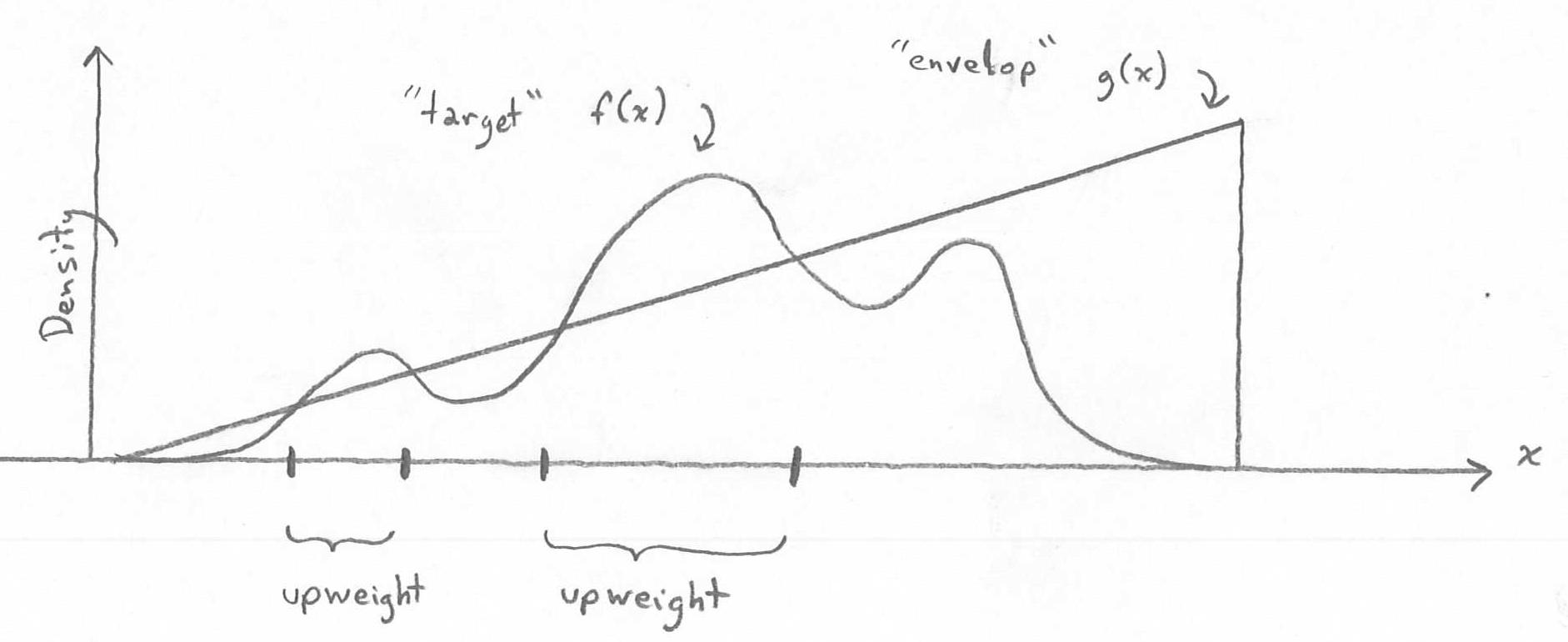Recall that, in rejection sampling, we either keep a draw from the envelop distribution (thereby accepting it as draw from the target distribution) or we reject it (thereby completely discarding it). Notationally, among the samples $$X^*_1,\ldots,X^*_{B^*}$$ from the envelop distribution, only a subset of them form the sample $$X_1,\ldots,X_B$$. We then do Monte Carlo integration on $$X_1,\ldots,X_B$$ to approximate $$\theta = E(h(X))$$: $\hat{\theta} = \frac{1}{B} \sum_{i=1}^B h(x_i).$ But we can rewrite this estimate in terms of the original samples $$X^*_1,\ldots,X^*_{B^*}$$ from the envelop distribution: $\hat{\theta} = \frac{1}{B^*} \sum_{i=1}^{B^*} w(x^*_i) h(x^*_i),$ where $$w(x^*_i) = 0$$ if $$X^*_i$$ is rejected and $$w(x^*_i) = B^*/B$$ otherwise. The $$\{w(x^*_i)\}_{i=1}^n$$ are called weights.

But why be so rigid in our weights? Why not permit weights other then $$0$$ and $$B^*/B$$? That is the idea behind importance sampling.Importance sampling diagram showing envelop function and target density.

Importance sampling accepts all the draws from the envelop distribution and gives them the weight $$w(x) = f(x)/g(x)$$, where $$f$$ and $$g$$ are the densities of the target and envelop distributions, respectively. The function $$w(x)$$ is called the weighting function. $\theta = E_f(h(X)) = \int h(x) f(x) dx = \int h(x) \frac{f(x)}{g(x)} g(x) dx = \int h(x) w(x) g(x) dx = E_g(h(X)w(X)) \approx \frac{1}{B^*} \sum_{i=1}^{B^*} h(x^*_i) w(x^*_i) = \hat{\theta},$ where $$x^*_1,\ldots,x^*_{B^*}$$ are all the samples from the envelop distribution. The standard error for a confidence interval to assess the Monto Carlo error in estimation of $$\theta$$ is: $\sqrt{ \frac{1}{B^*-1} \sum_{i=1}^{B^*} \big( h(x^*_i) w(x^*_i) - \hat{\theta} \big)^2 } \Bigg/ \sqrt{B^*}.$ Note that the Monte Carlo error can be very large if the weights have high variance.

### Unnormalized Density

What if the target density $$f$$ can only be evaluated up to a normalizing constant? That is, $$f(x) = c f^*(x)$$ and, yes, we can evaluate $$f^*(x)$$, but we do not know $$c$$ such that $$\int f(x) dx = \int c f^*(x) dx = 1$$. In this case, we can compute $$w^*(x) = f^*(x)/g(x)$$ even though we cannot compute $$w(x) = f(x)/g(x) = c f^*(x)/g(x) = c w^*(x)$$.

But we can obtain a Monte Carlo estimate of $$c$$: $c = \Big( \frac{1}{c} \Big)^{-1} = \Big( \frac{1}{c} \int c f^*(x) dx \Big)^{-1} = \Big( \int f^*(x) dx \Big)^{-1} = \Big( \int \frac{f^*(x)}{g(x)} g(x) dx \Big)^{-1} = \Big( \int w^*(x) g(x) dx \Big)^{-1} \approx \Big( \frac{1}{B^*} \sum_{i=1}^{B*} w^*(x^*_i) \Big)^{-1} = \hat{c}.$ Note that, by the Law of Large Numbers, $$\hat{c} \rightarrow_p c$$. Therefore, for fixed $$x$$, the estimated weighting function $\hat{w}(x) = \hat{c} w^*(x) = \frac{B^* w^*(x)}{\sum_{i=1}^{B^*} w^*(x^*_i)}.$ converges in probability to $$w(x)$$. Using these estimated weights, the Monte Carlo estimate of $$\theta$$ can be approximated by: $\hat{\theta} = \frac{1}{B^*} \sum_{i=1}^{B^*} h(x^*_i) w(x^*_i) \approx \frac{1}{B^*} \sum_{i=1}^{B^*} h(x^*_i) \hat{w}(x^*_i).$ Likewise the standard error for a confidence interval to assess the Monto Carlo error in estimation of $$\theta$$ is approximately: $\sqrt{ \frac{1}{B^*-1} \sum_{i=1}^{B^*} \big( h(x^*_i) \hat{w}(x^*_i) - \hat{\theta} \big)^2 } \Bigg/ \sqrt{B^*}.$